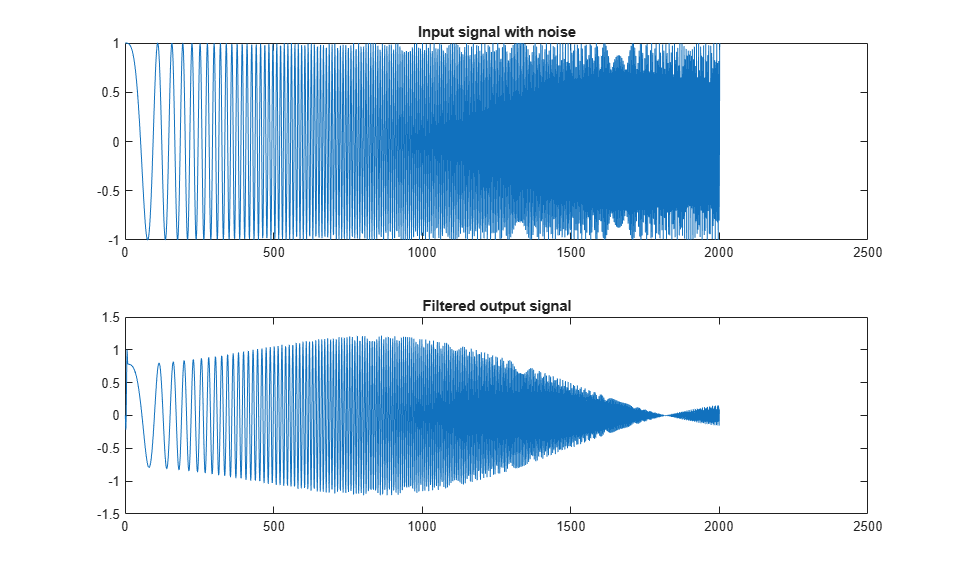# HDL Code Generation from System Objects

This example shows how to generate HDL code from MATLAB® code that contains System objects. For more information on System objects, see HDL Code Generation for System Objects.

### MATLAB Design

The MATLAB code used in this example implements a simple symmetric FIR filter and uses the `hdl.Delay` System object to model state. This example also shows a MATLAB test bench that exercises the filter.

```design_name = 'mlhdlc_sysobj_ex'; testbench_name = 'mlhdlc_sysobj_ex_tb'; ```

Look at the MATLAB design.

```type(design_name); ```
```%%%%%%%%%%%%%%%%%%%%%%%%%%%%%%%%%%%%%%%%%%%%%%%%%%%%%%%%%%%%%%%%%%%%%%%%%% % MATLAB design: Symmetric FIR Filter % % Design pattern covered in this example: % Filter states modeled using HDL System object (hdl.Delay) % Filter coefficients passed in as parameters to the design %%%%%%%%%%%%%%%%%%%%%%%%%%%%%%%%%%%%%%%%%%%%%%%%%%%%%%%%%%%%%%%%%%%%%%%%%% % Copyright 2011-2015 The MathWorks, Inc. %#codegen function [y_out, delayed_xout] = mlhdlc_sysobj_ex(x_in, h_in1, h_in2, h_in3, h_in4) % Symmetric FIR Filter persistent h1 h2 h3 h4 h5 h6 h7 h8; if isempty(h1) h1 = hdl.Delay; h2 = hdl.Delay; h3 = hdl.Delay; h4 = hdl.Delay; h5 = hdl.Delay; h6 = hdl.Delay; h7 = hdl.Delay; h8 = hdl.Delay; end h1p = step(h1, x_in); h2p = step(h2, h1p); h3p = step(h3, h2p); h4p = step(h4, h3p); h5p = step(h5, h4p); h6p = step(h6, h5p); h7p = step(h7, h6p); h8p = step(h8, h7p); a1 = h1p + h8p; a2 = h2p + h7p; a3 = h3p + h6p; a4 = h4p + h5p; m1 = h_in1 * a1; m2 = h_in2 * a2; m3 = h_in3 * a3; m4 = h_in4 * a4; a5 = m1 + m2; a6 = m3 + m4; % filtered output y_out = a5 + a6; % delayout input signal delayed_xout = h8p; end ```
```type(testbench_name); ```
```%%%%%%%%%%%%%%%%%%%%%%%%%%%%%%%%%%%%%%%%%%%%%%%%%%%%%%%%%%%%%%%%%%%%%%%%%% % MATLAB test bench for the FIR filter %%%%%%%%%%%%%%%%%%%%%%%%%%%%%%%%%%%%%%%%%%%%%%%%%%%%%%%%%%%%%%%%%%%%%%%%%% % Copyright 2011-2015 The MathWorks, Inc. clear mlhdlc_sysobj_ex; x_in = cos(2.*pi.*(0:0.001:2).*(1+(0:0.001:2).*75)).'; h1 = -0.1339; h2 = -0.0838; h3 = 0.2026; h4 = 0.4064; len = length(x_in); y_out_sysobj = zeros(1,len); x_out_sysobj = zeros(1,len); a = 10; for ii=1:len data = x_in(ii); % call to the design 'sfir' that is targeted for hardware [y_out_sysobj(ii), x_out_sysobj(ii)] = mlhdlc_sysobj_ex(data, h1, h2, h3, h4); end figure('Name', [mfilename, '_plot']); subplot(2,1,1); plot(1:len,x_in); title('Input signal with noise'); subplot(2,1,2); plot(1:len,y_out_sysobj); title('Filtered output signal'); ```

### Simulate the Design

Simulate the design with the test bench prior to code generation to make sure there are no runtime errors.

```mlhdlc_sysobj_ex_tb ```### Create a New HDL Coder™ Project

To create a new project, enter the following command:

```coder -hdlcoder -new mlhdlc_sysobj_prj ```

Next, add the file `mlhdlc_sysobj_ex.m` to the project as the MATLAB Function and `mlhdlc_sysobj_ex_tb.m` as the MATLAB Test Bench.

For a more complete tutorial on creating and populating MATLAB HDL Coder projects, see Get Started with MATLAB to HDL Workflow.

### Run Fixed-Point Conversion and HDL Code Generation

Launch the Workflow Advisor. In the Workflow Advisor, right-click the Code Generation step. Choose the option Run to selected task to run all the steps from the beginning through HDL code generation.

Examine the generated HDL code by clicking the links in the log window.

### Supported System objects

For a list of System objects supported for HDL code generation, see Predefined System Objects Supported for HDL Code Generation.